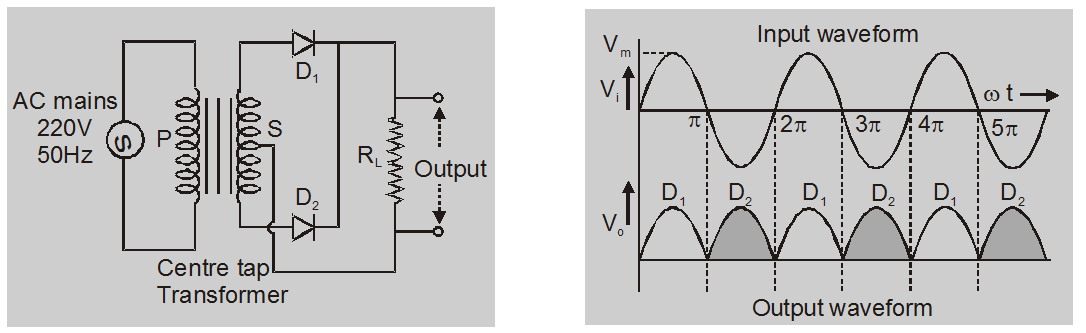Most Affordable JEE | NEET | 8,9,10 Preparation by Kota's Top IITian Doctor Faculties

# Full wave rectifier circuit diagram - Definition, Ripple Factor - eSaralA device that rectifies both halves of the ac input is called a full-wave rectifier. If you want to learn about the full-wave rectifier and full-wave rectifier circuit diagram then keep reading.

## Full-wave rectifier:

A rectifier that rectifies both halves of the ac input is called a full-wave rectifier.During the first half of the input cycle, the upper end of S coil is at positive potential and the lower end is at the negative potential the junction diode $\mathrm{D}_{1}$ will get forward biased, while the diode $\mathrm{D}_{2}$ reverse biased. The conventional current due to the diode $\mathrm{D}_{1}$ will flow.

When the second half of the input cycle comes, the situation will be exactly reverse. Now, the junction diode $\mathrm{D}_{2}$ will conduct and the current will flow.

## The efficiency of rectifier:

The efficiency rectifier is defined as the ratio of dc output power to the ac input power

$\eta=\frac{\text { dc power delivered to the load }}{\text { ac input power from transformer sec ondary }}$

$=\frac{P_{d c}}{P_{a c}}$

$=\frac{\mathrm{I}^{2} \mathrm{dc} \mathrm{R}_{\mathrm{L}}}{\mathrm{I}_{\mathrm{rms}}^{2}\left(\mathrm{R}_{\mathrm{F}}+\mathrm{R}_{\mathrm{L}}\right)}$

for Full wave rectifier

$\eta=\frac{.812}{1+\frac{R_{\mathrm{F}}}{R_{\mathrm{L}}}}$

if $\frac{R_{F}}{R_{L}}<<1$

$\eta=81.2 \%$

## Ripple and Ripple factor:

AC components are present in rectifier output these are known as ripple and they are measured in a factor which is known as Ripple Factor

Total Current Output

$\mathrm{I}_{\mathrm{rms}}=\sqrt{\mathrm{I}_{\mathrm{ac}}^{2}+\mathrm{I}^{2} \mathrm{dc}}$

Ripple Factor $=r=\frac{I_{\mathrm{ac}}}{\mathrm{I}_{\mathrm{dc}}}$

$r=\sqrt{\left(\frac{I_{r m s}}{I_{d c}}\right)^{2}-1}$

For full-wave or bridge rectifier

$\mathrm{I}_{\mathrm{rms}}=\frac{\mathrm{I}_{\mathrm{m}}}{\sqrt{2}}$

$I_{\mathrm{dc}}=\frac{2 \mathrm{I}_{\mathrm{m}}}{\pi}$

$r=0.48$

So, that's all from this article. I hope you get the idea about the full-wave rectifier and full-wave rectifier circuit diagram. If you have any confusion related to this blog then feel free to ask in the comments section down below.